# Weber function

(diff) ← Older revision | Latest revision (diff) | Newer revision → (diff)

The function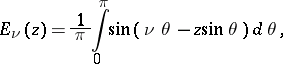whereis a complex number andis a real number. It satisfies the inhomogeneous Bessel equation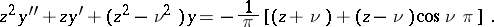For non-integralthe following expansion is valid: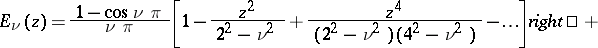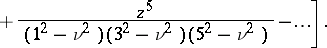Ifand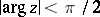, the following asymptotic expansion is valid: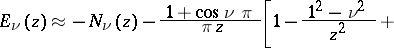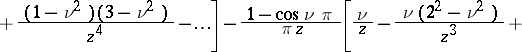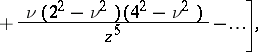where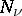is the Neumann function. Ifis not an integer, the Weber function is related to the Anger functionby the following equations: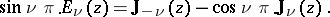The Weber functions were first studied by H. Weber .

How to Cite This Entry:
Weber function. Encyclopedia of Mathematics. URL: http://encyclopediaofmath.org/index.php?title=Weber_function&oldid=13892
This article was adapted from an original article by A.P. Prudnikov (originator), which appeared in Encyclopedia of Mathematics - ISBN 1402006098. See original article